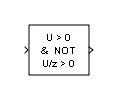# Detect Rise Positive

Detect rising edge when signal value increases to strictly positive value, and its previous value was nonpositive

• Library:
• Simulink / Logic and Bit Operations

•## Description

The Detect Rise Positive block detects a rising edge by determining if the input is strictly positive, and its previous value was nonpositive.

• The output is true (equal to `1`) when the input signal is greater than zero, and the previous value was less than or equal to zero.

• The output is false (equal to `0`) when the input is negative or zero, or if the input is positive, the previous value was also positive.

This block supports only discrete sample times.

## Ports

### Input

expand all

Input signal, specified as a scalar, vector, or matrix.

Data Types: `single` | `double` | `int8` | `int16` | `int32` | `int64` | `uint8` | `uint16` | `uint32` | `uint64` | `Boolean` | `fixed point`

### Output

expand all

Output signal that detects a rising edge whenever the input is strictly positive, and its previous value was nonpositive. The output can be a scalar, vector, or matrix.

• The output is true (equal to `1`) when the input signal is greater than zero, and the previous value was less than or equal to zero.

• The output is false (equal to `0`) when the input is negative or zero, or if the input is positive, the previous value was also positive.

Data Types: `uint8` | `Boolean`

## Parameters

expand all

Set the initial condition of the Boolean expression ```U/z > 0```.

#### Programmatic Use

 Block Parameter: `vinit` Type: character vector Values: scalar | vector | matrix Default: `'0'`

Specify whether the block performs sample- or frame-based processing:

• `Columns as channels (frame based)` — Treat each column of the input as a separate channel (frame-based processing).

Note

Frame-based processing requires a DSP System Toolbox™ license.

• `Elements as channels (sample based)` — Treat each element of the input as a separate channel (sample-based processing).

Use Input processing to specify whether the block performs sample- or frame-based processing. For more information about these two processing modes, see Sample- and Frame-Based Concepts (DSP System Toolbox).

#### Programmatic Use

 Block Parameter: `InputProcessing` Type: character vector Values: ```'Columns as channels (frame based)'``` | ```'Elements as channels (sample based)'``` Default: ```'Elements as channels (sample based)'```

Specify the output data type as `boolean` or `uint8`.

#### Programmatic Use

 Block Parameter: `OutDataTypeStr` Type: character vector Values: `'boolean' | 'uint8'` Default: `'boolean'`

## Block Characteristics

 Data Types `Boolean` | `double` | `fixed point` | `integer` | `single` Direct Feedthrough `yes` Multidimensional Signals `yes` Variable-Size Signals `yes` Zero-Crossing Detection `no`

## Version History

Introduced before R2006a